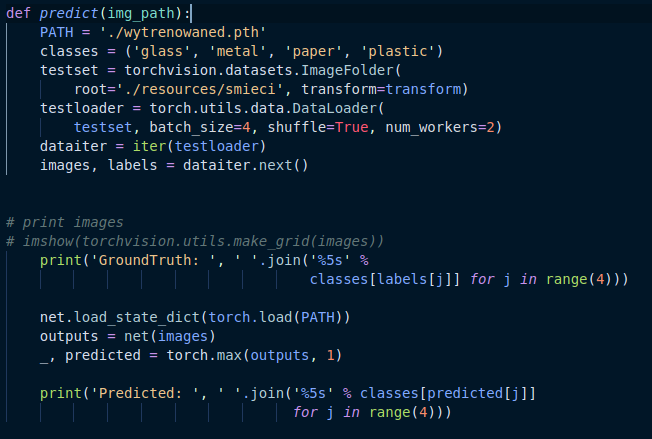# How do I pass single jpg file as an argument to predicting function in pytorch?

Hello,
i have started to work with pytorch in order to use it in my AI classes project. I have been following ‘60 minutes blitz’ tutorial and everything went quite smooth. The only difference is that im using ImageFolder - not the CIFAR10 dataset. Training my model went great but i occured a problem trying to modify an existing code. Previously, i used a folder with given classes(‘glass’,‘metal’,‘paper’,‘plastic’). My predicting function though needs to take an image path as an argument(just because i need to predict 1 single jpg)Can you please give me some tips on how do i convert the given code so it makes usage of img_path and returns the single guess?

I would like to add that i have tried to resolve it myself but during the process i have stumbled on such errors as
TypeError: only integer tensors of a single element can be converted to an index’,
IndexError: index 3 is out of bounds for dimension 0 with size 3

I would really appreciate some help, thank you

I have tried to convert the image to PIL and then transform it to tensor:

``````def predict(img_path):
PATH = './wytrenowaned.pth'
img = Image.open(img_path)
pil_to_tensor = transforms.ToTensor()(img).unsqueeze_(0)
print(pil_to_tensor)
classes = ('glass', 'metal', 'paper', 'plastic')
# testset = torchvision.datasets.ImageFolder(
#     root='./resources/smieci', transform=transform)
#     testset, batch_size=4, shuffle=True, num_workers=2)
# images, labels = dataiter.next()

# print images
# imshow(torchvision.utils.make_grid(images))
# print('GroundTruth: ', ' '.join('%5s' %
#                                 classes[labels[j]] for j in range(4)))
# print('---')
# print(images)
# print('---')
outputs = net(pil_to_tensor)
_, predicted = torch.max(outputs, 0)
``````

but it gives me an error

``````RuntimeError: Given groups=1, weight of size [6, 3, 5, 5], expected input[1, 1, 299, 299] to have 3 channels, but got 1 channels instead
``````

any ideas?

do you add 1 for the first dimension to your input tensor? its shape should be [1, c, h, w]. you can easily read the image and convert it to tensor and feed it into the net by the shape I mentioned.

i made it print out the shape of created tensors everytime function executes in my program and it goes like this:

``````torch.Size([1, 3, 299, 299])
torch.Size([1, 3, 299, 299])
torch.Size([1, 3, 299, 299])
torch.Size([1, 3, 299, 299])
torch.Size([1, 3, 299, 299])
torch.Size([1, 1, 299, 299])
Traceback (most recent call last):
File "main.py", line 11, in <module>
main()
File "main.py", line 7, in main
game.game()
outputs = net(pil_to_tensor)
result = self.forward(*input, **kwargs)
x = self.pool(F.relu(self.conv1(x)))
result = self.forward(*input, **kwargs)
return self._conv_forward(input, self.weight)
RuntimeError: Given groups=1, weight of size [6, 3, 5, 5], expected input[1, 1, 299, 299] to have 3 channels, but got 1 channels instead
``````

It looks like it has 1 for the first dim and 3 channels too. At some point channel changes from 3 to 1. Maybe it is something with provided images. Im not really sure what to do now

your input shape is [1, 1, 299, 299]? If so, it should be [1, 3, 299, 299]. You can first test your network with a random tensor to become sure if it works and then use the image with exact shape.

I’m not sure what are this printed shapes. Can you explain more?

Sure,
heres the code of printed tensors

``````def predict(img_path):
PATH = './wytrenowaned.pth'
img = Image.open(img_path)
pil_to_tensor = transforms.ToTensor()(img).unsqueeze_(0)
print(pil_to_tensor.shape)
``````

And my Net code goes as follows:

``````class Net(nn.Module):
def __init__(self):
super(Net, self).__init__()
self.conv1 = nn.Conv2d(3, 6, 5)
self.pool = nn.MaxPool2d(2, 2)
self.conv2 = nn.Conv2d(6, 16, 5)
self.fc1 = nn.Linear(16 * 71 * 71, 120)
self.fc2 = nn.Linear(120, 84)
self.fc3 = nn.Linear(84, 10)

def forward(self, x):
x = self.pool(F.relu(self.conv1(x)))
x = self.pool(F.relu(self.conv2(x)))
x = x.view(x.size(0), 16 * 71 * 71)
x = F.relu(self.fc1(x))
x = F.relu(self.fc2(x))
x = self.fc3(x)
return x
``````

i would like to point out that everything went smoothly while i was training and testing it on datasets created from my ImageFolder

It seems your model expect an image to have 3 channels but you are feeding an image with one channel. The printed shapes are different. 5 of them have 3 channel and one has 1 channel. So the network should work for first 5 images and not for the 6th image, right?

Yes and i think this is the case. Is it caused by some of the images though?It looks like guesses on those 3 channel tensors are just fine until the error with ‘1 channel one’ occures

yes. your image is gray. Maybe you can stack your image three times and feed it into your net.

1 Like

Thats exactly what i thought! I will find the path to the gray img since my dataset is rather small and give you some feedback! Thanks Isaac

1 Like

I made it work, the whole project is done. Thanks again!

1 Like